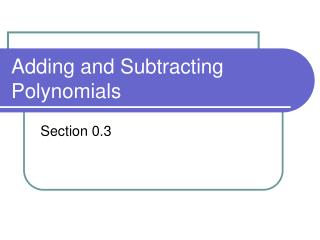DownloadDownload PresentationTélécharger la présentation- - - - - - - - - - - - - - - - - - - - - - - - - - - E N D - - - - - - - - - - - - - - - - - - - - - - - - - - -
Presentation Transcript

1. Adding and Subtracting Polynomials Section 0.3

2. Polynomial • A polynomial in x is an algebraic expression of the form: • The degree of the polynomial is n (largest exponent) • The leading coefficient is ( the coefficient on term with highest exponent) • The constant term is (the term without a variable) • The polynomial should be written in standard form. (Decreasing order according to exponents)

3. Polynomials Degree: 4 Leading Coefficient: 3 Constant: -9

4. Polynomials • Naming a polynomial: • 1 term - monomial • 2 terms - binomial • 3 terms - trinomial • 4 or more - terms polynomial • Example • 2x + 7 has 2 terms so it is called a binomial

5. 4 (a) 2 t + 7 2 2 2 (b) 3 e + 5 e – 9 e 2 = – e Classifying Polynomials The polynomial cannot be simplified. Two terms. The degree is 4. The polynomial is a binomial. The polynomial can be simplified. The degree is 2. The simplified polynomial is a monomial. One term.

6. Combine like terms and put the polynomial in standard form. What degree is the polynomial? Name the polynomial by the number of terms. Degree is 5 Trinomial

7. 4 3 4 3 Add 2n – 7n – 4 and – 5n – 8n + 10. 4 3 4 3 ( 2n – 7n – 4 ) + ( – 5n – 8n + 10 ) 3 4 – 15n – 3n Adding Polynomials Adding Polynomials Horizontally = + 6

8. 3 14y 2 – 5y Adding Polynomials 3 2 3 2 Find the sum (8y – 7y – y + 3) + (6y + 2y – 4y + 1). – 5y + 4

9. Subtracting Polynomials To subtract two polynomials, change all the signs of the second polynomial and add the result to the first polynomial. Subtracting Polynomials (Distribute the negative)

10. Subtracting Polynomials Perform the subtraction ( 3x – 5 ) – ( 6x – 4 ). ( 3x – 5 ) – ( 6x – 4 ) = 3x – 5 – 6x+ 4 = – 3x – 1 Change the signs in the second polynomial.

11. 2 2 2 2 ( 2ab – 4ab + b ) – ( 5ab – 3ab + 7b ) 2 2 = – 3a b – 6b Subtracting Multivariable Polynomials Add or subtract as indicated. 2 2 2 2 = 2ab – 4ab + b – 5a b + 3ab – 7b – ab

12. 2 4 (a) 5x ( 6x + 7 ) + 2 4 2 = 5x ( 6x ) 5x ( 7 ) 6 2 = 30x + 35x Multiplying Polynomials Use the distributive property to find each product. Distributive property Multiply monomials.

13. 4 9 2 (b) – 2h ( – 3h + 8h – 1 ) Multiplying Polynomials Use the distributive property to find each product.

14. 2 = 27g – 12g + 18g – 8 2 = 27g + 6g – 8 Multiplying Binomial times Binomial F O I L ( 3g + 2 ) ( 9g – 4 ) Multiply the First terms: 3g ( 9g ) F Multiply the Outer terms: 3g ( – 4 ) O Multiply the Inner terms: 2 ( 9g ) I Multiply the Last terms: 2 ( – 4 ) L

15. 2 2 = 24a – 6b Multiplying Polynomials ( 6a + 3b ) ( 4a – 2b )

16. 3 2 Multiply ( 2y – 5 )( 2y – 7y + 4 ). 3 2 ( 2y – 5 )( 2y – 7y + 4 ) (–7y) (4) + + = 2 2 2 3 3 (2y ) (2y ) (2y ) (2y ) (2y ) (–5) (–5) (–7y) (–5) (4) + + + 3 5 5 3 3 2 2 10y 35y 35y 4y 4y 14y 24y 8y 8y – – + + – + + = = – 20 Multiplying Binomial times Trinomial (Megafoil) Distributive property – 20 Combine like terms.

17. Square a binomial (x+4)² (x+4)(x+4) x² + 4x + 4x + 16 x² + 8x + 16 (x-7)² (x-7)(x-7) x² - 7x - 7x + 49 x² - 14x + 49

18. Square the binomial (2a-3b)² (2a-3b)(2a-3b) 4a² - 6ab - 6ab + 9b² 4a² - 12ab + 9b²

19. Find the product (x+7)(x-7) x² - 7x + 7x – 49 x² - 49 (2x - ½)(2x + ½) 4x² + x – x - ¼ 4x² - ¼

20. Simplify as much as possible -(2x – 6)² -(2x – 6) (2x – 6) -(4x² - 12x – 12x + 36) -(4x² - 24x + 36) -4x² + 24x – 36 3(2x – 4y)² 3(2x – 4y) (2x – 4y) 3(4x² - 8xy – 8xy + 16y²) 3(4x² - 16xy + 16y²) 12x² - 48xy + 48y²

21. Cubing a Binomial (x + 4)³ = (x + 4) (x + 4) (x + 4) = (x + 4)(x² + 8x + 16) = x(x²) + x(8x) + x(16) + 4(x²) + 4(8x) + 4(16) = x³ + 8x² + 16x + 4x² + 32x + 64 = x³ + 12x² + 48x + 64

22. Cubing a Binomial (2x – 3)³ (2x – 3)(2x – 3)(2x – 3) (2x – 3)(4x² - 12x + 9) 2x(4x²) + 2x(-12x) + 2x(9) – 3(4x²) – 3(-12x) – 3(9) 8x³ - 24x² + 18x – 12x² + 36x – 27 8x³ - 36x² + 54x – 27